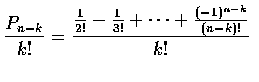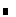The Matching Problem 配對理論

Example (Matching)
At a party n men take off their hats. The hats are then mixed up, and each man randomly selects one. We say that a match mixed up, and each man randomly selects one. We say that a mathc occurs if a man selects his own hat.
(a) What is probability of no matches?
(b) What is probability of exactly k matches?
Solution:
(a)
Let E denote the event that no matches occur, and to make explicit the dependence on n write Pn=P(E). We start by conditioning on whether or not the first man selects his own hat -- call these events M and Mc. Then Pn=P(E)=P(E|M)P(M)+P(E|Mc)P(Mc). Clearly, P(E|M)=0, so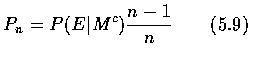Now, P(E|Mc) is the probability of no matches when n-1 men select from a set of n-1 hats that does not contain the hat of one of these men. This can happen in either of two mutually exclusive ways. Either there are no matches and the extra man does not select the extra hat (this being the hat of the man that chose first), or there are no matches and the extra man does select the extra hat. The probability of the first of these events is just Pn-1, which is seen by regarding the extra hat as "belonging" to the extra man. As the second event has probability [1/(n-1)]Pn-2, we have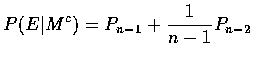and thus, from Equation (5.9),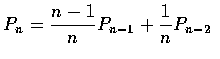or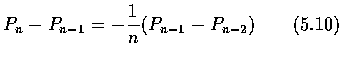However, as Pn is the probability of no matches when n men select among their own hats, we have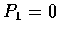,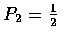so, from Equation (5.10),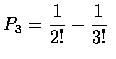and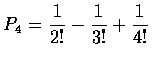and, in general, we see that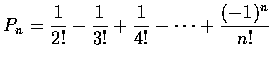(b)
To obtain the probability of exactly k matches, we consider any fixed group of k men. The probability that they, and only they, select their own hats is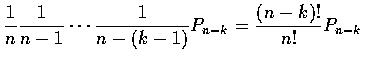where Pn-k is the conditional probability that the other n-k men, selecting among their own hats, have no matches. As there are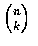choices of a set of k men, the desired probability of exactly k matches is